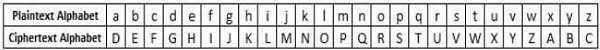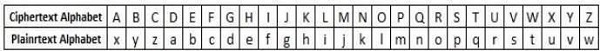# C++ Program to Implement Caesar Cypher

It is a mono-alphabetic cipher wherein each letter of the plaintext is substituted by another letter to form the ciphertext. It is a simplest form of substitution cipher scheme.

This cryptosystem is generally referred to as the Shift Cipher. The concept is to replace each alphabet by another alphabet which is ‘shifted’ by some fixed number between 0 and 25.

For this type of scheme, both sender and receiver agree on a ‘secret shift number’ for shifting the alphabet. This number which is between 0 and 25 becomes the key of encryption.

The name ‘Caesar Cipher’ is occasionally used to describe the Shift Cipher when the ‘shift of three’ is used.

## Process

• In order to encrypt a plaintext letter, the sender positions the sliding ruler underneath the first set of plaintext letters and slides it to LEFT by the number of positions of the secret shift.

• The plaintext letter is then encrypted to the ciphertext letter on the sliding ruler underneath. The result of this process is depicted in the following illustration for an agreed shift of three positions. In this case, the plaintext ‘tutorial’ is encrypted to the ciphertext ‘wxwruldo’. Here is the ciphertext alphabet for a Shift of 3 −• On receiving the ciphertext, the receiver who also knows the secret shift, positions his sliding ruler underneath the ciphertext alphabet and slides it to RIGHT by the agreed shift number, 3 in this case.

• He then replaces the ciphertext letter by the plaintext letter on the sliding ruler underneath. Hence the ciphertext ‘wxwruldo’ is decrypted to ‘tutorial’. To decrypt a message encoded with a Shift of 3, generate the plaintext alphabet using a shift of ‘-3’ as shown below −Here is the implementation of above process in C++.

## Steps and pseudocodes

Take the message and key as input −

### For encryption

• Input: tutorial.
• Output: wxwruldo

### For decryption

• Input: wxwruldo
• Output: tutorial

### For encryption

Begin
For i = 0 to msg[i] != '\0'
ch = msg[i]
//encrypt for lowercase letter
If (ch >= 'a' and ch <= 'z')
ch = ch + key
if (ch > 'z')
ch = ch - 'z' + 'a' - 1
done
msg[i] = ch
//encrypt for uppercase letter
else if (ch >= 'A' and ch <= 'Z')
ch = ch + key
if (ch > 'Z')
ch = ch - 'Z' + 'A' - 1
done
msg[i] = ch
done
done
Print Encrypted message
End

### For decryption

Begin
For i = 0 to msg[i] != '\0'
ch = msg[i]
//decrypt for lowercase letter
if(ch >= 'a' and ch <= 'z')
ch = ch - key
if (ch < 'a')
ch = ch +'z' - 'a' + 1
done
msg[i] = ch
//decrypt for uppercase letter
else if (ch >= 'A' and ch <= 'Z')
ch = ch + key
if (ch < 'A')
ch = ch + 'Z' - 'A' + 1
done
msg[i] = ch
done
done
Print decrypted message
End

## Example

#include<iostream>
#include<string.h>
using namespace std;
int main() {
cout<<"Enter the message:\n";
char msg;
cin.getline(msg,100); //take the message as input
int i, j, length,choice,key;
cout << "Enter key: ";
cin >> key; //take the key as input
length = strlen(msg);
cout<<"Enter your choice \n1. Encryption \n2. Decryption \n";
cin>>choice;
if (choice==1) //for encryption{
char ch;
for(int i = 0; msg[i] != '\0'; ++i) {
ch = msg[i];
//encrypt for lowercase letter
If (ch >= 'a' && ch <= 'z'){
ch = ch + key;
if (ch > 'z') {
ch = ch - 'z' + 'a' - 1;
}
msg[i] = ch;
}
//encrypt for uppercase letter
else if (ch >= 'A' && ch <= 'Z'){
ch = ch + key;
if (ch > 'Z'){
ch = ch - 'Z' + 'A' - 1;
}
msg[i] = ch;
}
}
printf("Encrypted message: %s", msg);
}
else if (choice == 2) { //for decryption
char ch;
for(int i = 0; msg[i] != '\0'; ++i) {
ch = msg[i];
//decrypt for lowercase letter
if(ch >= 'a' && ch <= 'z') {
ch = ch - key;
if(ch < 'a'){
ch = ch + 'z' - 'a' + 1;
}
msg[i] = ch;
}
//decrypt for uppercase letter
else if(ch >= 'A' && ch <= 'Z') {
ch = ch - key;
if(ch < 'A') {
ch = ch + 'Z' - 'A' + 1;
}
msg[i] = ch;
}
}
cout << "Decrypted message: " << msg;
}
}

## Output

For encryption:
Enter the message:
tutorial
Enter key: 3
1. Encryption
2. Decryption
1
Encrypted message: wxwruldo

For decryption:
Enter the message:
wxwruldo
Enter key: 3
Decrypted message: tutorial